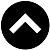-->

Type something and hit enter

# 你有听过72法则吗? 为什么是72? 不是71, 73?

## 神奇的72法则

time = 72/x
time = 72/20
time = 3.6 years

1 x 1.2 x 1.2 x 1.2 x 1.2 = 2.07

FV = PV x (1+x)^t

FV = Future Value
PV = Present Value
r = return
t = time

2PV = PV (1+r)^t
2 = (1+r)^t

ln(2) = t ln(1+r)
t = ln(2) / ln(1+r)

(别叫我解释)

t = ln(2)/r
t = 0.693/r

t = 69.3/r

https://klse.i3investor.com/blogs/calculatingcandlestickwinrate/2020-09-14-story-h1513493116.jsp